# kabuli

Showing the single result

• ## Dals & Pulses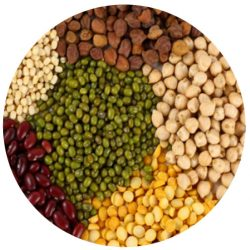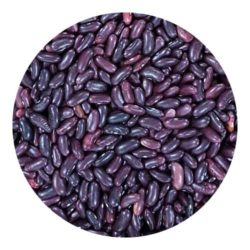27 Incl. Tax

Quick Order From our Dal and Pulses Menu, you can Blindly trust our quality. Soon you will realise why we say Accha Khao, Maze se Raho – Since 70 Years…..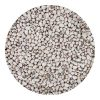Barbati Weight Choose Weight250gm - Rs. 27500gm - Rs. 501kg - Rs. 962kg - Rs. 1881.5kg - Rs. 1422.5kg - Rs. 2343kg - Rs. 280 Clear ₹27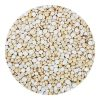Batana Daal Weight Choose Weight1kg - Rs. 922kg - Rs. 180500gm - Rs. 481.5kg - Rs. 1362.5kg - Rs. 2243kg - Rs. 268 Clear ₹48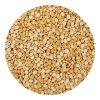Chana Daal Weight Choose Weight500gm - Rs. 481kg - Rs. 922kg - Rs. 1801.5kg - Rs. 1362.5kg - Rs. 2243kg - Rs. 268 Clear ₹48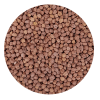Desi Chana Weight Choose Weight500gm - Rs. 431kg - Rs. 822kg - Rs. 1601.5kg - Rs. 1212.5kg - Rs. 1993kg - Rs. 238 Clear ₹43Daliya Weight Choose Weight250gm - Rs. 34500gm - Rs. 631kg - Rs. 1211.5kg - Rs. 1792.5kg - Rs. 2953kg - Rs. 353 Clear ₹34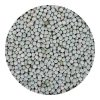Hara Batana Weight Choose Weight500gm - Rs. 571kg - Rs. 1101.5kg - Rs. 1632.5kg - Rs. 2693kg - Rs. 322 Clear ₹57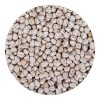Kabuli Chana Weight Choose Weight500gm - Rs. 1011kg - Rs. 1982kg - Rs. 3921.5kg - Rs. 2952.5kg - Rs. 4893kg - Rs. 586250gm - Rs. 53 Clear ₹53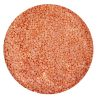Masoor Daal Weight Choose Weight200gm - Rs. 22500gm - Rs. 481kg - Rs. 922kg - Rs. 1801.5kg - Rs. 1362.5kg - Rs. 2243kg - Rs. 268 Clear ₹22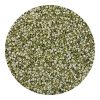Moong Daal Chilka Weight Choose Weight500gm - Rs. 661kg - Rs. 1282kg - Rs. 2521.5kg - Rs. 1902.5kg - Rs. 3143kg - Rs. 376 Clear ₹66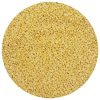Moong Mogar Weight Choose Weight500gm - Rs. 691kg - Rs. 1342kg - Rs. 2641.5kg - Rs. 1992.5kg - Rs. 3293kg - Rs. 394 Clear ₹69Moong Sabut Weight Choose Weight500gm - Rs. 641kg - Rs. 1242kg - Rs. 2441.5kg - Rs. 1842.5kg - Rs. 3043kg - Rs. 364250gm - Rs. 34 Clear ₹34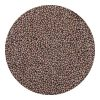Motha Weight Choose Weight500gm - Rs. 641kg - Rs. 1242kg - Rs. 2441.5kg - Rs. 1842.5kg - Rs. 3043kg - Rs. 364250gm - Rs. 34 Clear ₹34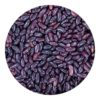Rajma Weight Choose Weight250gm - Rs. 44500gm - Rs. 841kg - Rs. 1641.5kg - Rs. 2442kg - Rs. 3243kg - Rs. 484 Clear ₹44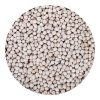Safed Batana Weight Choose Weight250gm - Rs. 24500gm - Rs. 441kg - Rs. 841.5kg - Rs. 1242.5kg - Rs. 2043kg - Rs. 244 Clear ₹24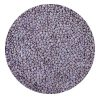Masoor Saiga Whole Weight Choose Weight250gm - Rs. 26500gm - Rs. 481kg - Rs. 921.5kg - Rs. 1362.5kg - Rs. 2243kg - Rs. 268 Clear ₹26Urad Saiga Whole Weight Choose Weight250gm - Rs. 35500gm - Rs. 661kg - Rs. 1281.5kg - Rs. 1902.5kg - Rs. 3143kg - Rs. 376 Clear ₹35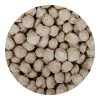Soyabean Badi Weight Choose Weight100gm - Rs. 14200gm - Rs. 24250gm - Rs. 29300gm - Rs. 34500gm - Rs. 54 Clear ₹14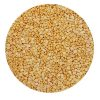Tur Daal Supreme Weight Choose Weight500gm - Rs. 981kg - Rs. 1922kg - Rs. 3801.5kg - Rs. 2862.5kg - Rs. 4743kg - Rs. 5685kg - Rs. 944 Clear ₹98Urad Daal Chilka Weight Choose Weight250gm - Rs. 36500gm - Rs. 681kg - Rs. 1321.5kg - Rs. 1962.5kg - Rs. 3243kg - Rs. 388 Clear ₹36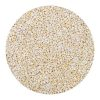Urad Daal Mogar Weight Choose Weight250gm - Rs. 40500gm - Rs. 761kg - Rs. 1482kg - Rs. 2921.5kg - Rs. 2202.5kg - Rs. 364 Clear ₹40

## Delete or Select Product from Collection by Selecting Pack type and Changing Quantity.

MRP: N/A SKU: wb3008808 Category: